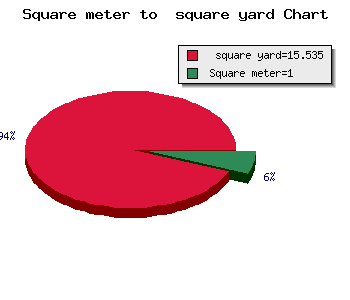×

# Square Meters to Square YardsConversions from one unit of measurement to another have always been fun. Remember your mathematics cases in school when your teacher asks how many cm (centimetres make a metre), how do you convert km to metres, etc.? Though mind-boggling, it kept your grey matter flowing and gave you an insight into the numerous standard units used in calculating area, volume etc. A square meter to square yards is yet another interesting conversion which we will discuss here.

What is a Square Yard?

A square yard is the unit of measurement which is used to calculate the area of land. A Square yard is widely used to calculate the area in countries like the US, Canada, UK, India and Pakistan. Denoted by sq yd or yd2 square yard is calculated using the following formula:

Length* Width to find the area of a property

This area calculated is divided by 9 to convert it onto square yards.

For example: 16 feet (length) * 12 feet (breadth) =192 square feet

192/9=21.22 square yards.

What is a square meter?

A square meter is an area that is equal to the square, which is one metre on every side. Denoted by m2, the square metre is used to calculate the area of rooms, houses, land etc.

How to convert square metres to square yards?

1 yard=3 feet

1 sq yd= 3* 3 feet (9 sq ft)

To convert from sq ft to sq yd, divide the given area by 9.

Example: In case you have a plot of 189 sq ft, and you want to convert it into sq yards, then the calculation will be:

189/9= 21 sq yd.I am Adil Saif working with AddressOfChoice Realty Private Limited as a Digital Marketing Expert. I have 8 Year Experience in same field. I love to share blog and Article.

Real Estates

Pune

Bangalore

Chandigarh

Greater Noida